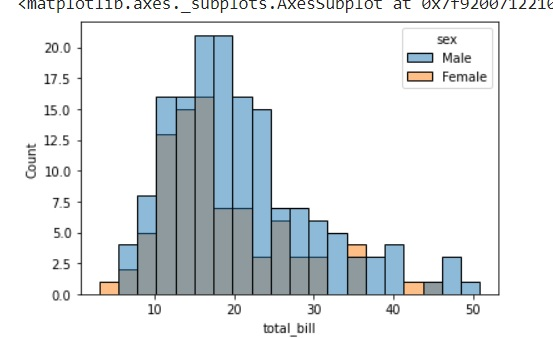Seaborn - Histogram

Histograms represent the data distribution by forming bins along the range of the data and then drawing bars to show the number of observations that fall in each bin.

Seaborn comes with some datasets and we have used few datasets in our previous chapters. We have learnt how to load the dataset and how to lookup the list of available datasets.

Seaborn comes with some datasets and we have used few datasets in our previous chapters. We have learnt how to load the dataset and how to lookup the list of available datasets.

Example

import pandas as pd
import seaborn as sb
from matplotlib import pyplot as plt
sb.distplot(df['petal_length'],kde = False)
plt.show()

OutputHere, kde flag is set to False. As a result, the representation of the kernel estimation plot will be removed and only histogram is plotted.

Useful Video Courses

Video

Data Visualization using MatPlotLib & Seaborn

11 Lectures 4 hours

Video

Seaborn with Python

11 Lectures 2.5 hours